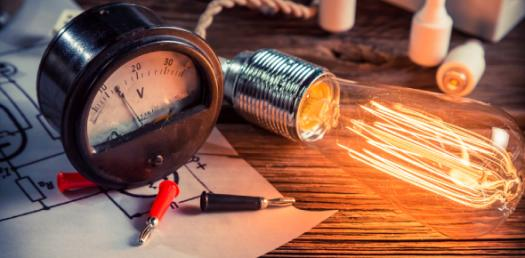# Physics Quiz: Effects Of Electric Current Trivia Questions!

13 Questions | Total Attempts: 2381Settings• 1.
Joule’s law of heating is (1)        (2)         (3)            (4)
• A.

(3)

• B.

(1)

• C.

(2)

• D.

(4)

• 2.
Nichrome wire is used as the heating element because it has
• A.

High specific resistance

• B.

Low melting point

• C.

Low specific resistance

• D.

High conductivity

• 3.
Peltier coefficient at a junction of a thermocouple depends on
• A.

The temperature of the junction

• B.

The charge that passes through the thermocouple

• C.

The time for which current flows

• D.

The current in the thermocouple

• 4.
In a thermocouple, the temperature of the cold junction is , the neutral temperature is . The temperature of inversion is
• A.

(1)

• B.

(2)

• C.

(3)

• D.

(4)

• 5.
Which of the following equations represents Biot-savart law? (1)         (2) (3)           (4)
• A.

(4)

• B.

(3)

• C.

(1)

• D.

(2)

• 6.
Magnetic induction due to an infinitely long straight conductor placed in a medium of permeability μ is
• A.

(4)

• B.

(2)

• C.

(3)

• D.

(1)

• 7.
In a tangent galvanometer, for a constant current, the deflection is . The plane of the coil is rotated through . Now, for the same current, the deflection will be
• A.

(4)

• B.

(2)

• C.

(1)

• D.

(3)

• 8.
The period of revolution of a charged particle inside a cyclotron does not depend on
• A.

The velocity of the particle

• B.

The magnetic induction

• C.

The charge of the particle

• D.

The mass of the particle

• 9.
The torque on a rectangular coil placed in a uniform magnetic field is large, when
• A.

the number of turns is large

• B.

The number of turns is less

• C.

The plane of the coil is perpendicular to the field

• D.

The area of the coil is small

• 10.
Phosphor – bronze wire is used for suspension in a moving coil galvanometer, because it has
• A.

Small couple per unit twist

• B.

High conductivity

• C.

Large couple per unit twist

• D.

High resistivity

• 11.
Of the following devices, which has small resistance?
• A.

Ammeter of range 0–10 A

• B.

Ammeter of range 0 – 1A

• C.

Voltmeter

• D.

Moving coil galvanometer

• 12.
A galvanometer of resistance G Ω is shunted with S Ω .The effective resistance of the combination is Ra. Then, which of the following statements is true?
• A.

Ra is less than both G and S

• B.

S is less than Ra but greater than G.

• C.

S is less than both G and Ra

• D.

G is less than S

• 13.
An ideal voltmeter has
• A.

infinite resistance

• B.

Zero resistance

• C.

Finite resistance less than G but greater than Zero

• D.

Resistance greater than G but less than infinity

Related TopicsBack to top